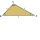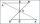Triangles

Ivo wants to draw all the triangles whose two sides of which have a length of 4 cm and 9 cm and the length of the third side is expressed in whole centimeters. How many triangles does he have?

Result

n =  7

Solution:Try calculation via our triangle calculator.

Leave us a comment of example and its solution (i.e. if it is still somewhat unclear...):Be the first to comment!To solve this example are needed these knowledge from mathematics:Five sticks with a length of 2,3,4,5,6 cm. How many ways can you choose three sticks to form three sides of a triangle?Hanka cut the 20 cm long straws into three pieces each piece had a length in cm. Then, with these three pieces, she tried to make a triangle. a) What circuit has each of the triangles? b) How long can the longest side measure? c) How many different trian